# Ratio + multiplication - math problems

#### Number of problems found: 54

• Three animals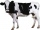Ramrao bought a cow, a buffalo and an ox for a total of rs 85,000. if the ratio of price of cow, buffalo and ox is 4: 8: 5, then what is the price of ox?
• The ratio 4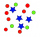The ratio of two number is 5:4 if 40% of the first number is 12, what will be 50% of the second number?
• Nails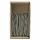If a keg of nails costs \$129.45 and 6/7 of the keg is used, what is the value of the remaining nails?
• Boys and girls 8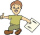In a class of 35 boys and girls that has a ratio of 2:5, 5 boys are absent and 5 boys are present. How many students are present?
• Boys and glasses2/3 of the students in Sarah’s class are boys. Of the boys, 1/3  of them wear glasses. What fraction of the students wear glasses?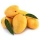A basket is full of 50 different kinds of fruits that has a ratio of 2:3:5. If the fruits are apple, mango, and guava, how many guavas are there?
• Boys and girlsIf the ratio of male & female teachers is 2/5 & it is in proportion of the male & female students, how many are girls if there are 42 boys?
• Dinner tickets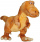At the spring festival, 7 out of the first 25 attendees had pre-purchased their dinner tickets. Based on this information, if 600 people attended the spring festival, then how many attendees could be expected to have pre-purchased their dinner tickets?
• Anna usesAnna uses 8.1 pints of white paint and blue paint to paint her bedroom walls. 4/5 of this amount is white paint, and the rest is blue paint. How many pints of blue paint did she use to paint her bedroom walls? Express your answer in fraction and decimal f
• Recipe 2Anna's making muffins! These ingredients make 12 muffins: 3/4 cup of flour 1/2 cup of sugar 1/4 teaspoon salt 1 cup of milk 1/3 teaspoon cinnamon 7 tablespoons butter 2 teaspoons baking powder 1 egg 2 tablespoons brown sugar Anna has a 8 muffin tray. What
• The kennel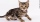20 dogs. Ratio dogs to cats is 3:2  how many cats?
• The ratioThe ratio of girls to boys in Mrs. White's class is 3:2.  If there are 12 boys, how many girls are in the classroom?
• Prime divisorsFind 2/3 of the sum's ratio and the product of all prime divisors of the number 120.
• Two xeroxes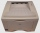The performances of the two copiers are in the ratio 3: 4. A machine with higher power will make 7,200 copies in one hour. How many copies will both machines make together in 5 hours?
• The plan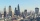The plan of the housing estate is in three scales 1: 5000,1: 10000,1: 15000. The distance between two points on a plan with a scale of 1: 10000 is 12 cm. What is this distance on the other two plans? What is this distance?
• Sum and ratioThe sum and ratio of two numbers is equal to 10. Which numbers are they?
• Apple juice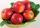From 7 kg of apples we get 3 liters of apple juice. How many liters of juice do we get from 42 kg of apples?
• Chicks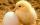How many chicks were hatched from 4500 eggs, when an average of 100 eggs hatched 87 chicks?
• Ratio - proportion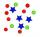Reduce the number 16 in proportion 3:2 5:4 11:8
• Change the numbers in the ratio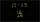Change the numbers 29, 38, and 43 in a 3: 4 ratio.

Do you have an exciting math question or word problem that you can't solve? Ask a question or post a math problem, and we can try to solve it.

We will send a solution to your e-mail address. Solved examples are also published here. Please enter the e-mail correctly and check whether you don't have a full mailbox.

Check out our ratio calculator. Ratio - math problems. Multiplication Problems.